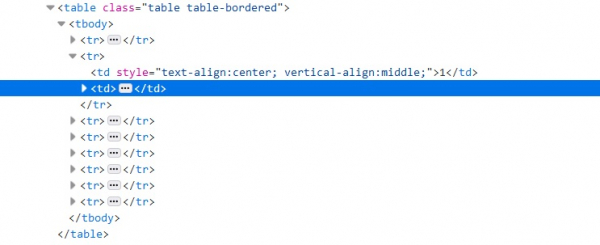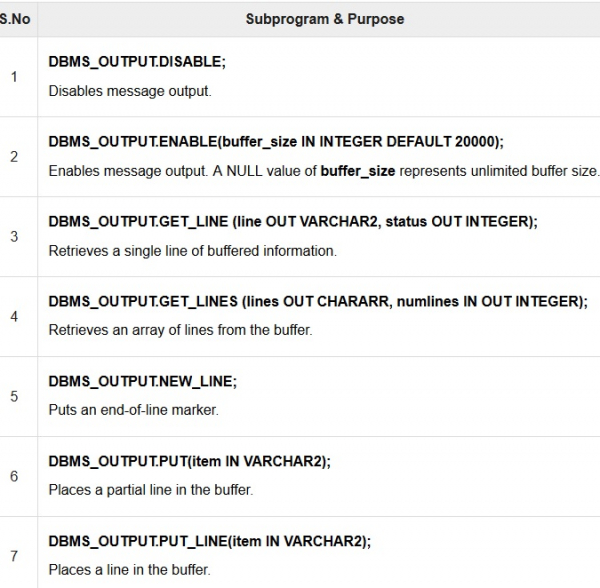# Handling Web Tables with Cypress

Web tables can be handled with Cypress. A web table can be of two types − static and dynamic. A static web table has a fixed number of rows and columns. A dynamic web table on the other hand has rows and columns whose numbers do not remain fixed.

In order to identify a particular column value in a table we need the help of css selector. A table structure has an html consisting of <table> tag followed by <tr> and finally <td> tag. The rows are represented by <tr> and the column values are represented by <td>.

With the help of the nth-child concept in css, we can identify all the values of a particular column. For example, for a table having two columns, in order to get all the values of the first column, the css should be tr td:nth-child(1). The structure of an html for table is shown below −Now to retrieve a value from a cell appearing in the next column of the same row, we have to move to the second column. In Cypress, we have the command next() to move to the immediate following sibling in DOM. The next() command works only if it is chained with get() command.

## Syntax

.next() , without arguments.
.next(selector/options), with argument.
next(selector, options), with arguments.

There can be of two types of options as listed below which can modify the default behavior of .next() command −

• log − The default value of log is true.

• timeout − The default value of timeout parameter is defaultCommandTimeout (4000 milliseconds). This the duration to wait for .next() to conclude.

## Example

Code Implementation to handle web tables.

describe('Tutorialspoint Test', function () {
// test case
it('Test Case4', function (){
cy.visit("https://www.tutorialspoint.com/plsql/plsql_dbms_output.htm");
// identify the second column for all the rows
cy.get('.table.table-bordered > tbody > tr > td:nth-child(1)').each(($el, index,$list) => {
// grabbing all text from second column
const txt = \$el.text();
// checking the matching condition
if (txt.includes('1')){
// capturing the next sibling with the help of next() method
cy.get('.table.table-bordered > tbody > tr > td:nth-child(1)')
.eq(index).next().then(function(desc){
// capturing the text of next sibling
const rsl = desc.text();
//assertion to verify the text
expect(rsl).to.contains('DBMS_OUTPUT.DISABLE');
})
}
})
});
});

The web table used for the above code implementation −